# math

``max( a, b )``
 max "maximum" a a number b a number

The max( a, b ) function is used to compare two numbers `a` and `b`, and it returns the greater of the two numbers.For example, if we call `max(5, 8)`, the function will compare the two arguments and determine that `8` is the greater value. Therefore, it will return `8`.

``````greater = max(5,8)
print(greater)  --prints 8``````

Similarly, if we call `max(-3, 0)`, the function will return `0` since `0` is greater than `-3`.

``````greater = max(0,-3)
print(greater)  --prints 0``````251

11 Apr 2023

``min( a, b )``
 min "minimum" a a number b a number

The min( a, b ) function is used to compare two numbers `a` and `b`, and it returns the lesser of the two numbers.For example, if we call `min(4, 18)`, the function will compare the two arguments and determine that `4` is the lesser value. Therefore, it will return `4`.

``````lesser = min(4,18)
print(lesser)  --prints 4``````

Similarly, if we call `min(-5, 1)`, the function will return `-5` since `-5` is less than `1`.

``````lesser = min(1,-5)
print(greater)  --prints -5``````198

11 Apr 2023

``mid( a, b, c )``
 min "minimum" a a number b a number c a number

The mid(a, b, c) function is used to determine the number in the middle of three given numbers `a`, `b`, and `c`.For example, if we call `mid(10, 4, 20)`, the function will compare the three arguments and determine that `10` is the middle value. Therefore, it will return `10`.

``````middle = mid(10, 4, 20)
print(middle)  --prints 10``````

#### Using mid() to Create Limits

One way to use this function in your game, is to make sure a number stays within a certain range of numbers. For example, keeping a player within the screen's width. The player's x coordinate can be limited to the screen's left side of 0 and right side of 127.

If we call `mid()`, with the arguments of the player x, and the minimum and maximum of the allowed range, then the player's x will be limited to those minimum and maximum values. Here's an example:

``````left=0
right=127
x=60 --player

function _update()
--player movement
if btn(⬅️) then x-=1 end
if btn(➡️) then x+=1 end

x= mid(left,right,x)
end

function _draw()
spr(player_sprite,x,120)
end``````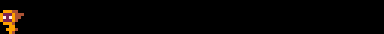Notice how the player does move off the right side of the screen. This is to demonstrate that you need to remember that a sprite's X and Y coordinates are for the top-left corner of the sprite. So using mid above is still working to limit the player's X on the screen, because it is not letting the player's left side leave the range of the screen.

To fix this, remember to adjust the right side limit by the sprite's width like so:

``x= mid(left,right-8,x)``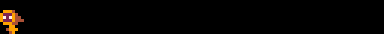251

4 Oct 2023

``flr( a )``
 flr "floor" a a number

The flr( a ) function is used to round down the given number, `a`, and return an integer (whole number).For example, if we call `flr(1.99)`, the function will round down to the nearest whole number and return that value. Therefore, it will return `1`.

``````value = flr(1.99)
print(value)  --prints 1``````

If we pass a negative number, for example `flr(-5.3)`, the function will return `-6` since it is the nearest whole number down.

``````value = flr(-5.3)
print(value)  --prints -6``````

There are many reasons you will want to "floor" a number. One common use is with the `time()` function, which almost always returns a decimal value, but you may want to simply know the number of seconds passed without any decimal places.

``````function _draw()
cls()
millisec = time()
sec = flr( millisec )

print("time="..millisec,20,40,8)
print("floored="..sec,20,60,12)
end``````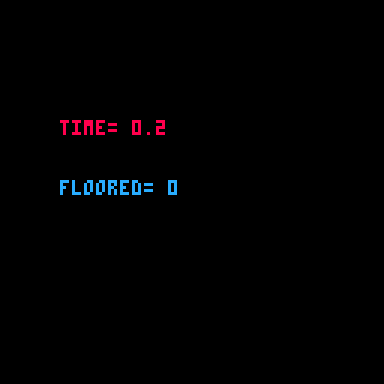#### Shorthand

It is common to want to floor a number after dividing, and there is a shorthand for that, the backslash ( `\` ).

``````--longform
a = flr(10/3)

--shorthand
b = 10\3``````

It is also common to want to floor a random number.

``````--longform
a = flr( rnd(10) ) --integer range 0-9

--shorthand
b = rnd(10)\1     --integer range 0-9``````243

12 May 2023

``ceil( a )``
 ceil "ceiling" a a number

The ceil( a ) function is used to round up the given number, `a`, and return the nearest larger whole number.For example, if we call `ceil(4.02)`, the function will round up to the nearest whole number and return that value. Therefore, it will return `5`.

``````value = flr(4.02)
print(value)  --prints 5``````

If we pass a negative number, for example `flr(-8.6)`, the function will return `-8` since it is the nearest larger whole number.

``````value = flr(-8.6)
print(value)  --prints -8``````

There are many reasons you may want to use `ceil()`. For example, in games that involve damage, health, or currency, you might want to ensure that these values are always rounded up to the nearest whole number. For example, if a character takes `3.2` points of damage, you might use `ceil()` to round that up to `4` points of damage because of a small powerup.211

22 Nov 2023

``cos( x )``
 cos "cosine" x a number

The cos( x ) function is used to return the cosine of the number provided.

An example from the manual shows us how to draw a rotating dial using cos() and sin():

``````function _draw()
cls()
circ(64, 64, 20, 7)
x = 64 + cos(t()) * 20
y = 64 + sin(t()) * 20
line(64, 64, X, Y)
end``````We've slowed it down and added some more details to show what is happening here:

``````function _draw()
cls()
circ(64, 64, 20, 5)
x = 64 + cos(t()/5) * 20
y = 64 + sin(t()/5) * 20
line(64, 64, x, 64,8)  -- x-axis cosine
line(64, 64, 64, y,11) -- y-axis sine
line(64, 64, x, y,6)   -- main dial line
pset(x, y,12)          -- (x,y) point
end``````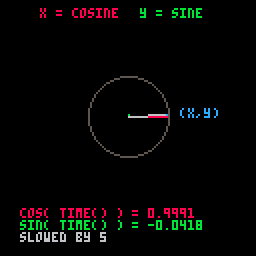263

4 Sep 2023

``sin( x )``
 sin "sine" x a number

The sin( x ) function is used to return the sine of the number provided.

An example from the manual shows us how to draw a rotating dial using cos() and sin():

``````function _draw()
cls()
circ(64, 64, 20, 7)
x = 64 + cos(t()) * 20
y = 64 + sin(t()) * 20
line(64, 64, X, Y)
end``````We've slowed it down and added some more details to show what is happening here:

``````function _draw()
cls()
circ(64, 64, 20, 5)
x = 64 + cos(t()/5) * 20
y = 64 + sin(t()/5) * 20
line(64, 64, x, 64,8)  -- x-axis cosine
line(64, 64, 64, y,11) -- y-axis sine
line(64, 64, x, y,6)   -- main dial line
pset(x, y,12)          -- (x,y) point
end``````256

4 Sep 2023

``atan2( dx, dy )``
 atan2 "2-argument arctangent" dx a number dy a number

The atan2(  ) function is used to return the angle of the numbers provided.

An example from the manual shows us how to get and draw the angle between two points using `atan2`:

``````X=20 Y=30
FUNCTION _UPDATE()
IF (BTN(0)) X-=2
IF (BTN(1)) X+=2
IF (BTN(2)) Y-=2
IF (BTN(3)) Y+=2
END

FUNCTION _DRAW()
CLS()
CIRCFILL(X,Y,2,14)
CIRCFILL(64,64,2,7)

A=ATAN2(X-64, Y-64)
PRINT("ANGLE: "..A)
LINE(64,64,
64+COS(A)*10,
64+SIN(A)*10,7)
END``````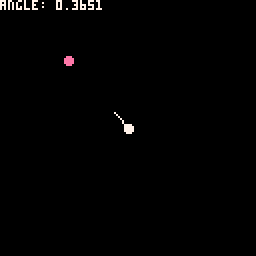We've added some more details to show what is happening here:

``angle = atan2( x1-x2, y1-y2 )``

Using the `angle` found with `atan2()` and combining it with `cos(angle)` and `sin(angle)`, we are able to draw the line that always points from one point to the other.267

4 Sep 2023

``sqrt( a )``
 sqrt "square root" a a number

The sqrt( a ) function is used to return the square root of the number provided.

For example, let's first square a number, then use sqrt() to reverse it:

``````--two ways to square a number
a = 4*4   --16
b = 4^2   --16

c = sqrt(b) --square root

print(c)  --4``````217

12 May 2023

``abs( a )``
 abs "absolute" a a number

The abs( a ) function is used to return the absolute number of the number given.

To understand what an absolute value is, imagine you are standing at any point on a number line and you want to know how far away from zero you are, no matter which side of zero you are standing (positive or negative). This is why the absolute value will always be a positive number.

For example:

``````a = abs(5)     --5
b = abs(-42)   --42

c = abs(3.75)  --3.45
d = abs(-6.25) --6.25``````232

12 May 2023

``rnd( a )``
 rnd "random" a a number or table

The rnd( a ) function is used to return a random number within the range of 0 up to but not including the number given.

#### Get Random Number

For example:

``````a = rnd(5)    --range: 0-4.9999
b = rnd(20)   --range: 0-19.9999
c = rnd(500)  --range: 0-499.9999``````

To get a random integer (whole number) use flr() with rnd() like this:

``````d = flr( rnd(10) )  --range: 0-9 integers

--shorthand floor
e = rnd(10)\1      --range: 0-9 integers``````

#### Get Random Table Value

You can also pass a table to the rnd() function to get a random value from the table:

``````table = { 4, 6, 8, 10 }

rnd(table)  --4, 6, 8, or 10``````

The table must have numbered keys because the function will find a random integer between 1 and the total count of the table and return the value stored at that table index. There is also a shorthand of providing the table itself in replace of the parentheses:

``````table={ 1, 3, 4, 10 }

--longform
table[ 1 + flr( rnd(#table) ) ]

--shorthand
rnd{ 1, 3, 4, 10 }``````

This can be useful for specifying a list of certain possible values, such as the spawn zone of an enemy, or the color numbers of a rectangle:

``````colors = { 8, 9, 12, 1, 4 }

rectfill( 40,50,60,70,rnd(colors) )
``````

Notice that the color numbers in the table can be in any order.328

12 May 2023

``srand( seed )``
 s (seed) a number to use as a seed value rand "random"

The `srand()` function is used to seed the random number generator: `rnd()`. In computer programming, a random number generator is not truly random, it is actually a deterministic algorithm that produces a sequence of seemingly random numbers. Every time a PICO-8 cart runs, the `rnd()` function uses a different seed value to begin generating its sequence of numbers.

By providing a seed value to `srand()`, you can control and reproduce the same sequence of random numbers.

For example:

``````--random every time
print( rnd(10) ) --unpredictable
print( rnd(10) ) --unpredictable
print( rnd(10) ) --unpredictable

--seeded random
srand(5)
print( rnd(10) ) --3.6121
print( rnd(10) ) --7.4159
print( rnd(10) ) --9.0311

--same seed, same results
srand(5)
print( rnd(10) ) --3.6121
print( rnd(10) ) --7.4159
print( rnd(10) ) --9.0311``````

This could be used with a random level generator to reproduce the same level or sequence of levels in your game.

Since `rnd()` can also be used to get values from tables at random, you can use `srand()` to make the table value selection more predictable and in a specific sequence.

``````table = { "a", "b", "c", "d" }

--random
print( rnd(table) ) --unpredictable

--seeded random
srand(5)
print( rnd(table) ) --a
print( rnd(table) ) --c
print( rnd(table) ) --d

--same seed, same results
srand(5)
print( rnd(table) ) --a
print( rnd(table) ) --c
print( rnd(table) ) --d``````284

19 Aug 2023

`Font`